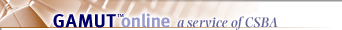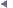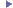Legal Resources | Education Code |  EC  11

Assessed values; tax rates; comparing different yearsPreviousNext(a) For purposes of this code, "assessed value" means 25 percent of full value to, and including, the 1980-81 fiscal year, and 100 percent of full value for the 1981-82 fiscal year and fiscal years thereafter; and, tax rates shall be expressed in dollars, or fractions thereof, on each one hundred dollars (\$100) of assessed value to, and including, the 1980-81 fiscal year and as a percentage of full value for the 1981-82 fiscal year and fiscal years thereafter.

(b) Whenever this code requires comparison of assessed values, tax rates or property tax revenues for different years, the assessment ratios and tax rates shall be adjusted as necessary so that the comparisons are made on the same basis, and the same amount of tax revenues would be produced, or the same relative value of an exemption or subvention will be realized regardless of the method of expressing tax rates or the assessment ratio utilized.

(c) For purposes of expressing tax rates on the same basis, a tax rate based on a 25 percent assessment ratio and expressed in dollars, or fractions thereof, for each one hundred dollars (\$100) of assessed value may be multiplied by a conversion factor of twenty-five hundredths of 1 percent to determine a rate comparable to a rate expressed as a percentage of full value; and, a rate expressed as a percentage of full value may be multiplied by a factor of 400 to determine a rate comparable to a rate expressed in dollars, or fractions thereof, for each one hundred dollars (\$100) of assessed value and based on a 25 percent assessment ratio.

(Added by Stats. 1978, Ch. 1207.)